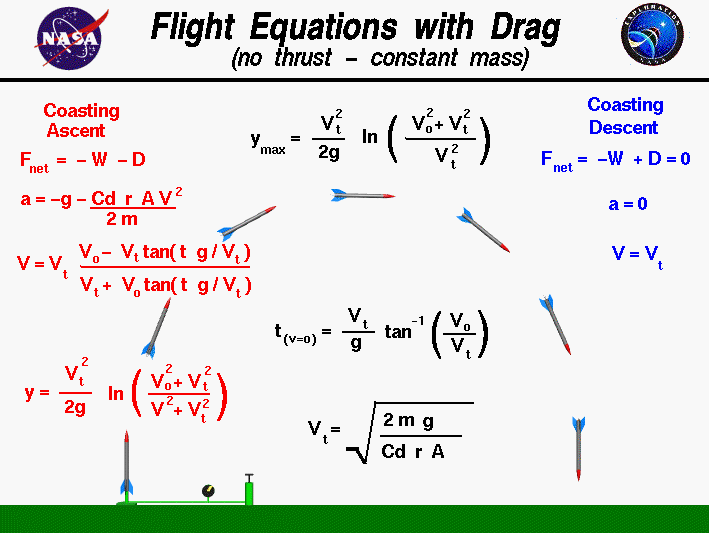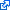+ Text Only Site
+ Non-Flash Version
+ Contact GlennThe study of rockets is an excellent way for students to learn the basics of forces and the response of an object to external forces. The easiest rocket to build and fly is the air rocket, which is often called a stomp rocket. The system uses an air pump to launch the rocket and the rocket coasts throughout the rest of the flight. Stomp rockets have no engine to produce thrust, so the resulting flight is similar to the flight of shell from a cannon, or a bullet from a gun. This type of flight is called ballistic flight and assumes that weight is the only force acting on the rocket. Stomp rockets generate a small amount of aerodynamic drag and are not strictly ballistic. On this page we develop the equations which describe the motion of a stomp rocket including the effects of drag. To simplify our analysis, we assume a perfectly vertical launch. If the launch is inclined at some angle, we resolve the initial velocity into a vertical and horizontal component. Unlike the ballistic flight equations, the horizontal equation includes the action of aerodynamic drag on the rocket. On this page, we assume that the horizontal force is much less than the vertical. For an object subject to only the forces of weight and drag, there is a characteristic velocity which appears in many of the equations. The characteristic velocity is called the terminal velocity because it is the constant velocity that the object sustains during the coasting descent. Terminal velocity is noted by the symbol Vt. During coasting descent, the weight and drag of an object are equal and opposite. There is no net force acting on the rocket and the vertical acceleration is zero. a = 0 W = D where a is the acceleration, W is the weight, and D is the drag. The weight of any object is given by the weight equation: W = m * g where m is the mass of the object and g is the gravitational acceleration equal to 32.2 ft/sec^2 or 9.8 m/sec^2 on the surface of the Earth. The gravitational acceleration has different values on the Moon and on Mars. The drag is given by the drag equation: D = .5 * Cd * r * A * Vt^2 where r is the gas density, Cd is the drag coefficient which characterizes the effects of shape of the rocket, A is the cross-sectional area of the rocket, and Vt is the terminal velocity. The gas density has different surface values on the Earth and on Mars and varies with altitude. On the Moon the gas density is zero. Combining the last three equations, we can determine the terminal velocity: m * g = .5 * Cd * r * A * Vt^2 Vt = sqrt ( (2 * m * g) / (Cd * r * A) ) Now, turning to the ascent trajectory, the rocket is traveling at an initial vertical velocity Vo. For the stomp rocket the velocity is set by the launch mechanism and there is no thrust once the rocket is launched. With the positive vertical coordinate denoted by y, the net vertical force Fnet acting on the rocket is given by: Fnet = -W -D Because the weight of the object is a constant, we can use the simple form of Newton's second law to solve for the vertical acceleration: Fnet = m a = -W - D m a = - (m * g) - (.5 * Cd * r * A * v^2) a = -g - (Cd * r * A * v^2) / (2 * m) Multiply the last term by g/g and use the definition of the terminal velocity to obtain: a = -g * (1 + v^2 / Vt^2) The acceleration is the time rate of change of velocity : a = dv/dt = -g * (1 + v^2 / Vt^2) Integrating this differential equation: dv / (1 + v^2 / Vt^2) = -g dt Vt * tan-1(v/Vt) = -g * t where tan-1 is the inverse tangent function, and t is time.. The limits of integration for velocity v is from Vo to V and the limits for time t is from 0 to t: tan-1(V/Vt) - tan-1(Vo/Vt) = - g * t / Vt tan-1(V/Vt) = tan-1(Vo/Vt) - g * t / Vt Now take the tangent function of both sides of the equation using the trigonometric identity: tan(a - b) = (tan(a) - tan(b))/(1 + tan(a)*tan(b)) on the right hand side to obtain: V/Vt = (Vo/Vt - tan(g * t / Vt)) / (1 + (Vo/Vt) * tan (g * t / Vt)) V/Vt = (Vo - Vt * tan(g * t / Vt)) / (Vt + Vo * tan (g * t / Vt)) This is the equation for the velocity at any time during the coasting ascent. At the top of the trajectory, the velocity is zero. We can solve the velocity equation to determine the time when this occurs: Vo/Vt = tan(g * t(v=o) / Vt) t(v=o) = (Vt / g) * tan-1(Vo/Vt) To determine the vertical location during the ascent, we have to use another identity from differential calculus: dv/dt = dv/dy * dy/dt dv/dt = v * dv/dy We previously determined that dv/dt = -g * (1 + v^2 / Vt^2) v * dv/dy = -g * (1 + v^2 / Vt^2) (v /(1 + v^2 / Vt^2)) * dv = -g dy Integrating both sides: (Vt^2 / 2) * (ln (v^2 + Vt^2)) = - g * y where ln is the natural logarithmic function. The limits of integration for velocity v is from Vo to V and the limits for direction y is from 0 to y: (Vt^2 / 2) * (ln (V^2 + Vt^2) - ln (Vo^2 + Vt^2) = - g * y y = (Vt^2 / (2 * g)) * ln ((Vo^2 + Vt^2)/(V^2 + Vt^2)) Notice that the location equation is pretty messy! For a given time t, we would have to find the local velocity V, and then plug that value into the location equation to get the location y. At the maximum height ymax, the velocity is equal to zero: ymax = (Vt^2 / (2 * g)) * ln ((Vo^2 + Vt^2)/Vt^2) Here's a Java calculator which solves the equations presented on this page: Due to IT security concerns, many users are currently experiencing problems running NASA Glenn educational applets. The applets are slowly being updated, but it is a lengthy process. If you are familiar with Java Runtime Environments (JRE), you may want to try downloading the applet and running it on an Integrated Development Environment (IDE) such as Netbeans or Eclipse. The following are tutorials for running Java applets on either IDE: NetbeansEclipseThis page shows an interactive Java applet which calculates the max altitude and the time which it occurs for a rocket subjected to weight and drag. To operate the calculator, you first select the planet using the choice button at the top left. For purely ballistic flight, select the "Ignore Drag" option with the middle choice button. For flight with drag, select "Include Drag" with the middle choice button. You can perform the calculations in English (Imperial) or metric units. Enter the initial velocity. Since we are performing the calculation with drag, we must specify the object's weight, cross sectional area, and a drag coefficient. The air density is determined by the altitude, or it can be input directly. Press the red "Compute" button to compute the maximum height and the time to maximum height. The program also outputs the terminal velocity as described above. We provide an on-line web page that contains only this calculator. You can also download your own copy of the calculator for use off-line. The program is provided as Fltcalc.zip. You must save this file on your hard drive and "Extract" the necessary files from Fltcalc.zip. Click on "Fltcalc.html" to launch your browser and load the program. You can also study the flight characteristics of a ballistic object with drag by using the on-line RocketModeler III simulation program. Notice If you toggle the middle choice button between "Ignore Drag" and "Include Drag", you will notice that the computed height is always less when including the drag. The amount of the difference indicates the importance of drag for certain flight conditions. Also consult the terminal velocity web page for some warnings concerning cases with high terminal velocity. If you hold the initial velocity constant, and increase only the weight, you will notice that the maximum height gradually approaches the ballistic flight value. Guided ToursCompressed Air Rocket:Water Rocket:Model Rocket:Flight Equations:RocketModeler III:Ballistic Flight Calculator :Activities: Related Sites: Rocket Index Rocket Home Beginner's Guide Home+ Inspector General Hotline + Equal Employment Opportunity Data Posted Pursuant to the No Fear Act + Budgets, Strategic Plans and Accountability Reports + Freedom of Information Act + The President's Management Agenda + NASA Privacy Statement, Disclaimer, and Accessibility CertificationEditor: Tom Benson NASA Official: Tom Benson Last Updated: Apr 06 2018 + Contact Glenn